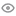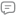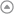New update is available. Click here to update.Graphic Era (Deemed to be University) 2026My StatsEXP gained12659Level
7 (Expert)
Community statsDiscussions
00
Know more195
Total problems solved
128
Easy
60
Moderate
7
Hard
0
NinjaCurrent streak:

0 days

Longest streak:

11 days

Less

More

Achievements5
Ronin
Topics
Binary Search
Strings
+ 3 more2
Samurai
Topics
Arrays
Binary Trees
Discussions
JAVA SOL || Boundary Traversal of Binary Tree
Interview problems

import java.util.ArrayList;

import java.util.List;

public class Solution {

// Function for obtaining LeafNodes-:

public static int LeafNodes(TreeNode root, List<Integer> ans){

if(root == null) return 0;

int lh = LeafNodes(root.left, ans);

int rh = LeafNodes(root.right, ans);

if(lh == rh && lh == 0) ans.add(root.data); // when both lh and rh are zero it means current node is leaf node

return 1;

}

//Function for printing right side outermost nodes -> printing nodes from bottom to up-:

public static void reverseOuterRight(TreeNode root, List<Integer> ans)

{

if(root.left == null && root.right == null) return;

if(root.right == null) reverseOuterRight(root.left, ans);

else reverseOuterRight(root.right, ans);

}

// Main Function-:

public static List<Integer> traverseBoundary(TreeNode root){

List<Integer> ans = new ArrayList<>();

// Outer left-:

TreeNode temp = root.left;      // starting from root's left node

if(root.left != null){          // if root's left is null it means there is no outer left nodes in tree.

while(temp.left != null || temp.right != null)

{

if(temp.left == null) temp = temp.right;    // if left is null go to right

else    temp = temp.left;                   // otherwise go always to left node

}

}

// Leaf nodes-:

LeafNodes(root, ans);

// Outer right-:

temp = root.right;          // starting from root's left node

if(root.right != null)          // if root's right is null it means there is no outer right nodes in tree.

{

reverseOuterRight(temp, ans);

}

return ans;

}

}

Luckyn1
Published On 21-Nov-202336 views0 repliesBIT MAINUPLATION || JAVA SOL.
Interview problems

public class Solution{

public static int missingNumber(int n, int []arr){

int ans = arr;

for(int i=1; i<n; i++){

ans = ans ^ arr[i];        // preforming 'XOR' in each element bqz of this twice element will remove and only number which occurs only one time will remains in n

}

return ans;

}

}

Luckyn1
Published On 28-Oct-202326 views0 repliesJava Sol || Bit Mainuplation || Swap two numbers
Interview problems

public class Solution{

public static void swapNumber(int []a, int []b) {

// let a = 5 => 101, b = 6 => 110

a = a ^ b;     // a = (5 ^ 6)

b = a ^ b;     // b = a ^ 6 = (5^6) ^ 6 => 5 [bqz 'XOR' of same no. is zero]

a = a ^ b;     // a = a ^ b = (5^6) ^ (5) => 6

}

}

Luckyn1
Published On 27-Oct-2023140 views0 repliesJAVA SOL || Delete All Occurences
Interview problems

public class Solution {

public static Node deleteAllOccurrences(Node head, int k) {

while(temp != null){

// if key found -: deleting element

if(temp.data == k)

{

// if there is only one node in list-:

if(temp.next == temp.prev) return null;

// if it is first node-:

else if(temp.prev == null)

{

}

// if it is last node-:

else if(temp.next == null) temp.prev.next = null;

// otherwise-:

else

{

temp.prev.next = temp.next;

temp.next.prev = temp.prev;

}

}

temp = temp.next;

}

}

}

Luckyn1
Published On 21-Sep-202362 views0 repliesOptimal Approach 2 || JAVA SOL.
Interview problems

public class Solution {

// traversing both LL until we find there intersection point

// if there is no intersection point so there will be a point when dumy1 = dumy2 = null

while(dumy1 != null && dumy2 != null)

{

if(dumy1 == dumy2) return dumy1.data;        // if dumy1 == dumy2 it means that node is the point of intersection

dumy1 = dumy1.next;

dumy2 = dumy2.next;

// if dumy1 is null but dumy2 is not, so point dumy1 to the head of second LL(secondHead)

if( dumy1 == null && dumy2 != null ) dumy1 = secondHead;

// if dumy2 is null but dumy1 is not, so point dumy2 to the head of first LL(firstHead)

if( dumy2 == null && dumy1 != null ) dumy2 = firstHead;

}

return 0;

}

}

Luckyn1
Published On 18-Sep-202395 views0 repliesOptimal Approach 1 || JAVA SOL.
Interview problems

public class Solution {

int l1 = 0, l2 = 0;

// finding length of both LL

while(dumy1 != null) {

l1++;

dumy1 = dumy1.next;

}

while(dumy2 != null){

l2++;

dumy2 = dumy2.next;

}

int l = l1 > l2 ? (l1 - l2) : (l2 - l1);     // finding differnce of lengths

int i = 0;

// if length of first LL is greater than second LL so fNode will tarverse to l nodes so when we compare both LL will be in same position

if(l1 > l2){

while(i < l){

fNode = fNode.next;

i++;

}

}

// if length of second LL is greater than first LL so sNode will tarverse to l nodes so when we compare both LL will be in same position

else{

while(i < l){

sNode = sNode.next;

i++;

}

}

// traversing LL

while(fNode != null){

if(fNode == sNode) return fNode.data;       // if we get both nodes are equal it means that node is the first intersection node

fNode = fNode.next;

sNode = sNode.next;

}

return 0;

}

}

Luckyn1
Published On 18-Sep-202349 views0 repliesJAVA SOL. || Sort LL of 0s 1s 2s || find and connect nodes
Interview problems

public class Solution

{

{

Node x = null;      // to connect 0's node only

Node y = null;      // to connect 1's node only

Node z = null;      // to connect 2's node only

Node xEntry = null;     // to store first 0's node

Node yEntry = null;     // to store first 1's node

Node zEntry = null;     // to store first 2's node

// traversing through LL-:

while(temp != null)

{

// connecting 0's nodes

if(temp.data == 0)

{

// if it is first 0's node

if(x == null)

{

x = temp;

xEntry = x;

}

else{

x.next = temp;

x = temp;

}

}

// connecting 1's nodes

else if(temp.data == 1){

if(y == null) {

y = temp;

yEntry = y;

}

else{

y.next = temp;

y = temp;

}

}

// connecting 2's nodes

else{

if(z == null){

z = temp;

zEntry = z;

}

else{

z.next = temp;

z = temp;

}

}

temp = temp.next;

}

// connecting 0's, 1's and 2's node with each other

x.next = yEntry;

y.next = zEntry;

z.next = null;

return xEntry;

}

}

Luckyn1
Published On 17-Sep-2023115 views0 repliesJAVA SOL. || Delete Node of LL
Interview problems

public class Solution {

public static Node deleteLast(Node list){

Node p = list;

//  if linkedlist is empty {there is zero node} or there is only one node -> we don't need to delete any node

if(p == null || p.next == null )

return list;

// Otherwise-:

// traversing until we reach to second last node-:

while(p.next.next != null){

p = p.next;

}

// making second last node's next = null [it means last node deleted]

p.next = null;

return list;

}

}

Luckyn1
Published On 10-Sep-2023162 views0 repliesJAVA SOL || two line code || Insert Node at the begining
Interview problems

public class Solution

{

public static Node insertAtFirst(Node list, int newValue) {

// creating new node which have data newValue and its next is pointing to the head of given linkedlist -:

}

}

Luckyn1
Published On 10-Sep-2023108 views0 repliesJAVA SOL || Introduction to LL
Interview problems

public class Solution {

public static Node constructLL(int []arr)

{

// with the help of this we will insert element in LinkedList and connect the nodes with the next node

Node LL =new Node();

// it will store access of first node

Node ans =LL;

for(int i=0; i<arr.length; i++)

{

// every time we make this node and store element in this than gave its address to LL which will connect the new node with LinkedList

Node p = new Node();

p.data = arr[i];

p.next = null;

// when LL is empty-:

if(i==0) {

ans = LL = p;

continue;

}

LL.next = p;

LL = p;

}

// when we inserted all element in LL so we are making last node's next null which indicates end of LL

if(LL != null) LL.next = null;

return ans;

}

}

Luckyn1
Published On 10-Sep-2023277 views0 replies